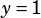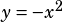A question from the May 2018 SAT (Section 4 #18)

kx + y = 1
y = -x² + k

In the system of equations above, k is a constant. When the equations are graphed in the xy-plane, the graphs intersect at exactly two points. Which of the following CANNOT be the value of k?

A. 3
B. 2
C. 1
D. 0

Because this comes in the calculator section, you might think that my recommendation is to graph them all. Indeed, if you go that route, you’ll get the answer, and it won’t take that long! However, if you notice that making k equal zero eliminates the x term in the first equation, you might not need to bother graphing anything else.

When, the equations becomeand. The former is just a horizontal line above the x-axis, and the latter is a downward-facing parabola that intersects the origin. Those will never intersect, so zero cannot be the value of k.